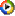Feb 26, 2016

Feb 26, 2016

Feb 26, 2016

# Principal Method of Terrestrial PhotogrammetryThe principal is exactly similar to plan table surveying, it may be stated as “The position of the object with ref to the base line is given by the intersection of the rays drawn to it form each end of the base line” In plane tabling most of the work is executed in the field while in this method it is done in the office. The principal is explained as follows

C and D = Camera stations.

CD = Base line of length ‘B’

CE and DE = Position of vertical plane of collimation. and

B = Observed vertical angles which the vertical plane of collimation make with base line at C and D.

M = pt to be located, which is shown as ‘m’ in both the photographs.

X1 and Y1 = Coordinates of ‘M’ on pt’C’Watch a Video on How to Apply Terrestrial Photogrammetry Method

X2 and Y2 = ----------------------------‘D’

F = focal length of camera lens(Photograph from ‘C’) (Photograph from ‘D’)

## Method of location PT ‘M’:

Pt ‘M’ may be plotted graphically or analytically.### Graphical Method :

• First plot the base line to the given scale
• Draw CE making an angles of ‘ ’ wide CD with the help of a protector.
• Similarly draw DE making an angle of B with BE.
• On CE mark the pt ’e’ at a distance equal to fine font of ‘C’. Similarly setoff a distance ‘De’ equal to ‘f’ along DE in front of D as shown.
• To these pts and ‘e’ draw lines right angles ot CE and DE respectively measure ‘em’ equal to X1and To X2 along these perpendicular lines on the same side as on the photographs. (Her they are measured on ht left of CD and DE)
• Join ‘CM’ and ‘DM’ and produce them so as to meet at ‘M’ which gives required position of ‘M’ on Plane

### To determine the level of PT ‘M’

• Measure ‘y’ which gives the height of ‘m’ above the horizontal hair. Rise of the ray from ‘m’ to the center of the object glass is equal to ‘y’ in a horizontal dist• Measure the distance ‘CM’ to the scale on the plane.
• The height ‘H’ of ‘M’ above the horizontal Plane of collimation at ‘C’ is given by Knowing the reduced level (RL) of horizontal plane of collimation, the RL of M may be obtained as R.L of M= R.L of horizontal plane of collimation at 'C' is given by:### Field Work:

The field work of terrestrial photographic surveying consists of

• Reconnaissance
• Triangulation
• Camera work